### Home > A2C > Chapter 4 > Lesson 4.2.1 > Problem4-82

4-82.
1. Factor each of the following expressions. Look for the difference of squares and common factors. Homework Help ✎

1. 4x2 − 9y2

2. 8x3 − 2x 7

3. x4 −81y4

4. 8x3 + 2x7

5. Did you use a shortcut to factor the expressions in parts (a) through (c)? If so, describe it. If not, what pattern do you see in these expressions? How can you use that pattern to factor quickly?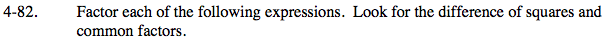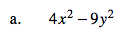Remember the rule (a + b)(ab) = (a2b2).

(2x + 3y)(2x − 3y)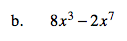Remember to remove common factors.

2x3(4 − x4)

2x3(2 − x2)(2+ x2)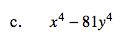See part (a).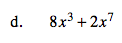See part (b).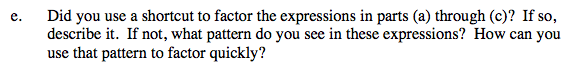The pattern for parts (a) through (c) is that they contain a difference of squares.